Open in App
Not now

# Image Recognition using TensorFlow

• Last Updated : 09 Oct, 2022

In this article, we’ll create an image recognition model using TensorFlow and Keras. TensorFlow is a robust deep learning framework, and Keras is a high-level API(Application Programming Interface) that provides a modular, easy-to-use, and organized interface to solve real-life deep learning problems.

## Image Recognition:

In Image recognition, we input an image into a neural network and get a label (that belongs to a pre-defined class) for that image as an output. There can be multiple classes for the labeled image. If it belongs to a single class, then we call it recognition; if there are multiple classes, we call it classification.

In this article, we use a flower dataset with 3670  images with five classes labeled as daisy, dandelion, roses, sunflowers, and tulips. The Image Classification model consists of the following steps:

• Understand data and load data: In this stage, we need to collect image data and label them. If the images are downloaded from other sources, then also they must be preprocessed before using them for training.
• Build input pipeline: Tensorflow APIs allow us to create input pipelines to generate input data and preprocess them effectively for the training process. The pipeline for an image model aggregates data from files in a distributed file system applies random perturbations to each image and merges randomly selected images into a batch for training.
• Build the model: In this stage, we make choices about parameters and hyperparameters and make decisions about the number of layers to be used in our model. We decide on the input and output sizes of the layers, along with the activation function.
• Train the model: After creating a model, we must create an instance of the model and fit it with our training data.
• Test the model: The crucial part of this stage is to estimate: the amount of time the model takes to train and specify the length of training for a network depending on the number of epochs to train over.
• Evaluate and improve the accuracy: Evaluating a model means comparing its performance against a validation dataset to analyze its performance through different metrics. The most common metric is ‘Accuracy’, calculated by dividing the amount of correctly classified images by the total number of images in our dataset.

### Step 1 : Importing TensorFlow and other libraries

The first step is to import the necessary libraries and modules such as pyplot, NumPy library, tensor-TensorFlow, os, and PIL.

## Python3

 `# Importing libraries``import` `matplotlib.pyplot as plt``import` `numpy as np``import` `os``import` `PIL``import` `tensorflow as tf`` ` `from` `tensorflow ``import` `keras``from` `tensorflow.keras ``import` `layers``from` `tensorflow.keras.models ``import` `Sequential`

## Python3

 `# importing flower dataset``import` `pathlib`` ` `dataset_url ``=` `"https:``/``/``storage.googleapis.com``/``download.\``tensorflow.org``/``example_images``/``flower_photos.tgz"``data_dir ``=` `tf.keras.utils.get_file(``    ``'flower_photos'``, origin``=``dataset_url, untar``=``True``)``data_dir ``=` `pathlib.Path(data_dir)`

Now, after downloading, we can count total images by using len() method. Here, glob() method is used to find jpg files in the specified directory recursively.

## Python3

 `image_count ``=` `len``(``list``(data_dir.glob(``'*/*.jpg'``)))``print``(image_count)`

Output:

`3670`

We can check that there are 3670 images in the given directory.

## Python3

 `roses ``=` `list``(data_dir.glob(``'roses/*'``))``PIL.Image.``open``(``str``(roses[``0``]))`

Output: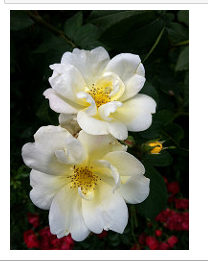The code given above will display an image of the rose. Here, PIL(Python Image Library )is used to display images.

### Step 3: Creating a model

To work with images, let’s load the images to our disk using tf.keras.utils.image_dataset_from_directory utility. We use a training split 80% of the images for training and 20% for validation when developing our model.

Training Split:

## Python3

 `# Training split``train_ds ``=` `tf.keras.utils.image_dataset_from_directory(``    ``data_dir,``    ``validation_split``=``0.2``,``    ``subset``=``"training"``,``    ``seed``=``123``,``    ``image_size``=``(``180``, ``180``),``    ``batch_size``=``32``)`

```Found 3670 files belonging to 5 classes.
Using 2936 files for training.```

Validation Split:

Here we are providing a testing dataset to split in an 80:20 ratio, just as we did in the training split in the above code.

## Python3

 `# Testing or Validation split``val_ds ``=` `tf.keras.utils.image_dataset_from_directory(``    ``data_dir,``    ``validation_split``=``0.2``,``    ``subset``=``"validation"``,``    ``seed``=``123``,``    ``image_size``=``(``180``,``180``),``    ``batch_size``=``32``)`

Output:

```Found 3670 files belonging to 5 classes.
Using 734 files for validation.```

We can check the class names by calling the class_names attribute on the training dataset in alphabetical order in the preceding code.

## Python3

 `class_names ``=` `train_ds.class_names``print``(class_names)`

Output:

`['daisy', 'dandelion', 'roses', 'sunflowers', 'tulips']`

Visualizing the datasets:

Here we have used pyplot module from matplotlib library to view our training dataset. We can view 25 images from training dataset.

## Python3

 `import` `matplotlib.pyplot as plt`` ` `plt.figure(figsize``=``(``10``, ``10``))`` ` `for` `images, labels ``in` `train_ds.take(``1``):``    ``for` `i ``in` `range``(``25``):``        ``ax ``=` `plt.subplot(``5``, ``5``, i ``+` `1``)``        ``plt.imshow(images[i].numpy().astype(``"uint8"``))``        ``plt.title(class_names[labels[i]])``        ``plt.axis(``"off"``)`

Output: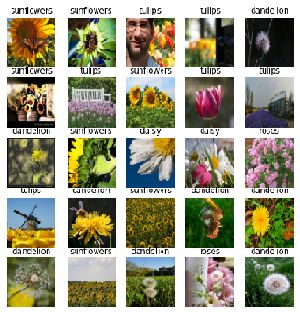Here, we have created a figure of size(10,10) using plt.figure() function and from using a for loop applied on the training dataset we arrange images using subplot() function and using imshow() function, we displayed the photos on the figure.

### Step 4: Creating the model:

Now it is the stage where we design the CNN (Convolutional Neural Network) model. Keras has numerous formats to build models, the Sequential model is the most commonly used model as it consists of three convolution layers (Conv2D, MaxPooling2D, and Dense) with 128 units on top of it is activated by a ReLU activation function(‘relu’). Let’s create model using Sequential() function.

## Python3

 `num_classes ``=` `len``(class_names)`` ` `model ``=` `Sequential([``    ``layers.Rescaling(``1.``/``255``, input_shape``=``(``180``,``180``, ``3``)),``    ``layers.Conv2D(``16``, ``3``, padding``=``'same'``, activation``=``'relu'``),``    ``layers.MaxPooling2D(),``    ``layers.Conv2D(``32``, ``3``, padding``=``'same'``, activation``=``'relu'``),``    ``layers.MaxPooling2D(),``    ``layers.Conv2D(``64``, ``3``, padding``=``'same'``, activation``=``'relu'``),``    ``layers.MaxPooling2D(),``    ``layers.Flatten(),``    ``layers.Dense(``128``, activation``=``'relu'``),``    ``layers.Dense(num_classes)``])`

Step 5: Compiling the model:

To view training and validation accuracy for each training epoch, pass the metrics argument to model.compile() method. Here we have used ‘adam’ optimizer and SparseCategoricalCrossentropy() loss function to evaluate the loss. Here we have used model.summary() method that allows us to view all the layers of the network.

## Python3

 `model.``compile``(optimizer``=``'adam'``,``              ``loss``=``tf.keras.losses.SparseCategoricalCrossentropy(``                  ``from_logits``=``True``),``              ``metrics``=``[``'accuracy'``])``model.summary()`

Output: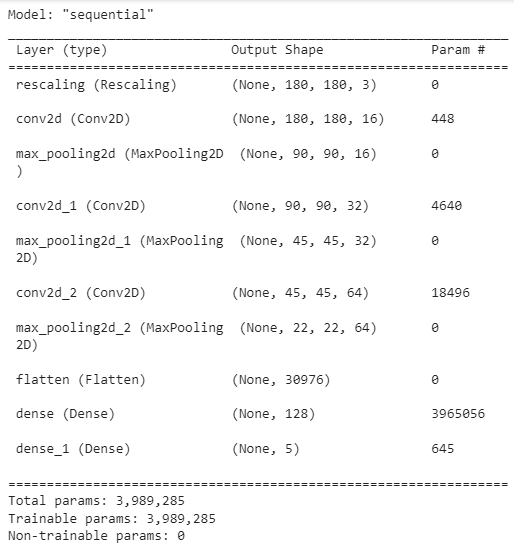Train the model using model.fit() method that allows the machine to learn patterns by providing training and test/validation dataset to the model.

## Python3

 `epochs``=``10``history ``=` `model.fit(``  ``train_ds,``  ``validation_data``=``val_ds,``  ``epochs``=``epochs``)`

Output :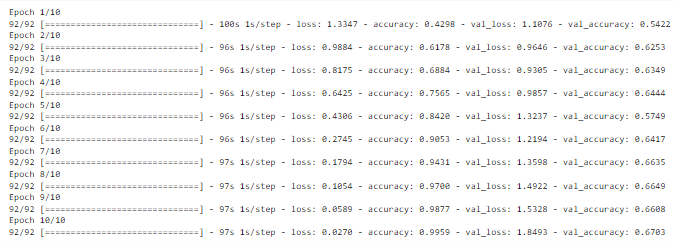With each epoch, accuracy is changed.

## Visualizing result on training dataset:

Creating plots of accuracy and loss on the training and validation sets to consider bias and variance.

## Python3

 `#Accuracy``acc ``=` `history.history[``'accuracy'``]``val_acc ``=` `history.history[``'val_accuracy'``]`` ` `#loss``loss ``=` `history.history[``'loss'``]``val_loss ``=` `history.history[``'val_loss'``]`` ` `#epochs ``epochs_range ``=` `range``(epochs)`` ` `#Plotting graphs``plt.figure(figsize``=``(``8``, ``8``))``plt.subplot(``1``, ``2``, ``1``)``plt.plot(epochs_range, acc, label``=``'Training Accuracy'``)``plt.plot(epochs_range, val_acc, label``=``'Validation Accuracy'``)``plt.legend(loc``=``'lower right'``)``plt.title(``'Training and Validation Accuracy'``)`` ` `plt.subplot(``1``, ``2``, ``2``)``plt.plot(epochs_range, loss, label``=``'Training Loss'``)``plt.plot(epochs_range, val_loss, label``=``'Validation Loss'``)``plt.legend(loc``=``'upper right'``)``plt.title(``'Training and Validation Loss'``)``plt.show()`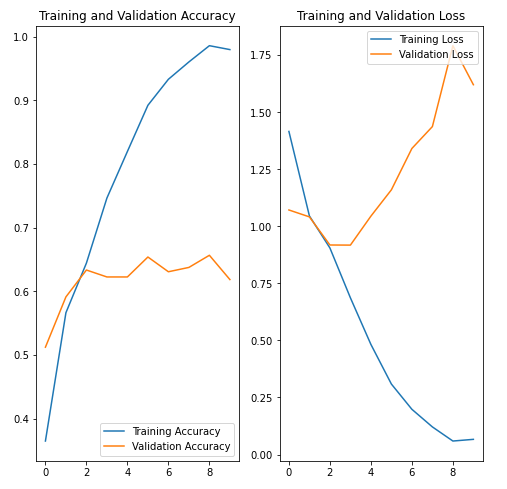Here the plots visualize that training accuracy and validation accuracy.

## Conclusion

Now we can have learnt that how to perform image recognition using TensorFlow.

My Personal Notes arrow_drop_up